## Indicators

•Cited by SciELO
•Access statistics

•Cited by Google
•Similars in SciELO
•Similars in Google

## Print version ISSN 0120-419XOn-line version ISSN 2145-8472

### Integración - UIS vol.39 no.1 Bucaramanga Jan./June 2021  Epub Feb 28, 2021

#### https://doi.org/10.18273/revint.v39n1-2021007

Artículos Originales

Some special types of determinants in graded skew PBW extensions*

3Universidad Nacional de Colombia, Departamento de Matemáticas

Abstract

In this paper, we prove that the Nakayama automorphism of a graded skew PBW extension over a finitely presented Koszul Auslanderregular algebra has trivial homological determinant. For A = σ(R) {x1, x2} a graded skew PBW extension over a connected algebra R, we compute its Pdeterminant and the inverse of σ. In the particular case of quasi-commutative skew PBW extensions over Koszul Artin-Schelter regular algebras, we show explicitly the connection between the Nakayama automorphism of the ring of coefficients and the extension. Finally, we give conditions to guarantee that A is Calabi-Yau. We provide illustrative examples of the theory concerning algebras of interest in noncommutative algebraic geometry and noncommutative differential geometry.

Keywords Calabi-Yau algebra; skew PBW extension; double Ore extension; homological determinant; P-determinant; Nakayama automorphism; MSC2010: 16S37; 16W50; 16W70; 16S36; 13N10

Resumen

En este artículo, demostramos que el automorfismo de Nakayama de una extensión PBW torcida graduada sobre un álgebra de Koszul finitamente presentada y Auslander-regular tiene determinante homológico trivial. Para A = σ(R) {x1, x2} una extensión PBW torcida graduada sobre un álgebra conexa R, calculamos su P-determinante y el inverso de σ. En el caso particular de extensiones PBW torcidas cuasi-conmutativas sobre álgebras de Koszul Artin-Schelter regulares, mostramos explícitamente la relación entre el automorfismo de Nakayama del anillo de coeficientes y la extensión. Finalmente, damos condiciones para garantizar que A sea Calabi-Yau. Proporcionamos ejemplos ilustrativos de la teoría con álgebras de interés en geometría algebraica no conmutativa y geometría diferencial no conmutativa.

Palabras clave Álgebra Calabi-Yau; extensión PBW torcida; extensión de Ore doble; determinante homológico; P-determinante; automorfismo de Nakayama

1. Introduction

In  the homological determinant was defined and used to study the Artin-Schelter regular property of some algebras. Shen et al.,  and Wu et al.,  gave equivalent definitions of the homological determinant and established connections between homological determinant and the usual determinant. On the other hand, Nakayama automorphism plays an important role in noncommutative algebraic geometry (see Reyes et al., ) and its computation is not easy in the general case. Some authors have computed and studied this automorphism for special types of algebras, see for example [22, 23, 24, 27, 33, 34, 39, 45]. The remarkable fact is the relationship between both notions, see Reyes et al., , Shen et al.,  and Zhun et al., , for more details. Double Ore extensions, denoted by A = RP [x1, x2; σ, δ, τ ], were defined by Zhang and Zhang  where they proved that a connected graded double Ore extension of an ArtinSchelter regular algebra is Artin-Schelter regular. Later, in , they constructed 26 families of Artin-Schelter regular algebras of global dimension four by using double Ore extensions. For R a Koszul Artin-Schelter regular algebra, Zhun et al.,  calculated the Nakayama automorphism of a trimmed double Ore extension RP [x1, x2; σ], and the Nakayama automorphism of an iterated skew polynomial extension of a Koszul ArtinSchelter regular algebra by using the homological determinant. Another properties of double Ore extensions have been explored by Carvalho et al.,  and Zhun et al., .

Now, for A = RP [x1, x2; σ, δ, τ ] a right double Ore extension, Zhang and Zhang  introduced the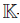-linear map det Pσ : R → R of σ called the P-determinant. This Pdeterminant was used to prove the Artin-Schelter regular property of double Ore extensions. Zhun et al.,  used the P-determinant to calculate the Nakayama automorphism of certain algebras. For the case where R is a Koszul Artin-Schelter regular algebra and σ : R → M2(R) is an algebra homomorphism, Zhu et al.,  defined the homological determinant of σ, denoted by hdetM, and presented some connections between the Nakayama automorphism, the homological determinant, and the P-determinant. These authors used the homological determinant of σ to know when a trimmed double Ore extension of a Koszul Artin-Schelter regular algebra turns out to be Calabi-Yau.

The noncommutative rings of polynomial type of interest in this paper are the skew P BW extensions, denoted by A = σ(R){x1, . . . , xn}, which were defined by Gallego and Lezama  with the aim of generalizing another families of noncommutative rings appearing in several branches of mathematics (c.f. Bell and Goodearl  and Ore ). These objects have been recently studied (e.g., [1, 2, 11, 12, 13, 17, 18, 19, 21, 40, 42]), and, as a matter of fact, a book containing some of the works developed for these objects has been published recently by Springer . As a particular class of these objects, Suárez  defined graded skew P BW extensions over an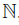-graded algebra R and showed that if R is a finitely presented Koszul algebra, then every graded skew PBW extension over R is Koszul. The Artin-Schelter regular property and the skew Calabi-Yau condition for graded skew PBW extensions were studied by Suárez et al., . There, the authors proved that every graded quasi-commutative skew PBW extension of an Artin-Schelter regular algebra is also Artin-Schelter regular; every graded quasi-commutative skew PBW extension over a connected skew Calabi-Yau algebra is skew Calabi-Yau; and every graded skew PBW extension over a connected Auslander regular algebras is Artin-Schelter regular and skew Calabi-Yau. With respect to the Nakayama automorphism, Suárez et al.,  described it for A = σ(R) {x1, . . . , xn} by using the Nakayama automorphism of an Artin-Schelter regular algebra R, and also they calculated explicitly the Nakayama automorphism of some skew PBW extensions. About doble Ore extensions, Gómez and Suárez  gave necessary and sufficient conditions for a graded (trimmed) double Ore extension to be a graded (quasi-commutative) skew PBW extension. They proved that graded skew PBW extensions A = σ(R) {x1, x2} over Artin-Schelter regular algebras R are also ArtinSchelter regular, and graded skew PBW extensions A = σ(R){x1, x2} over connected skew Calabi-Yau algebras R of dimension d are skew Calabi-Yau of dimension d + 2.

With all above results in mind, next, we present the structure of the article by mentioning the original results presented here. In Section 2, we present definitions and basic properties of ring theory and skew PBW extensions. In Section 3, we present some new results about these extensions. Briefly, Theorem 3.1 asserts that the Nakayama automorphism of a graded skew PBW extension A = σ(R){x1, . . . , xn} over a finitely presented Koszul Auslander-regular algebra R has trivial homological determinant. A particular case of this fact is presented in Corollary 3.2, where we show that the Nakayama automorphism of graded skew PBW extensions over the commutative polynomial ring R =[t1, . . . , tm] is equal to 1. Now, since a graded skew PBW extension over a connected algebra R is a connected graded double Ore extension RP [x1, x2; σ, δ, τ ], in Theorem 3.4 we calculate the P-determinant of σ and the inverse of σ. In Theorem 3.5, for a quasi-commutative Calabi-Yau skew PBW extension A = σ(R){x1, x2} = RP [x1, x2; σ] over a Koszul ArtinSchelter regular algebra, we calculate the Nakayama automorphism of R and the homological determinant of σ in terms of the definition of skew PBW extension. In this sense, we also show the correlation between the Nakayama automorphism, the homological determinant and the homological determinant of σ. Finally in Corollary 3.6, we calculate the Nakayama automorphism of a graded quasi-commutative skew PBW extension A = σ(R){x1, x2} over a Koszul Artin-Schelter regular algebra R with Nakayama automorphism, and we establish a sufficient and necessary condition to guarantee that A is Calabi-Yau. In this way, this paper continues the research about Koszul, CalabiYau, Artin-Schelter and related ring theoretic notions for skew PBW extensions (c.f. [29, 36, 37, 38, 39]).

2. Preliminaries

Throughout the paper, the word ring means an associative ring with identity not necessarily commutative. If B is a ring, Mn(B) denotes the set of matrices of size n × n with entries in B.denotes a field; all algebras are-algebras. The symbol N will be used to denote the set of natural numbers including zero, and the tensor product ⊗ means ⊗K. An algebra B is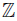-graded, if there exists a family of subspaces {Bp}p∈Z of B such that B =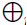p∈Z Bp and BpBq ⊆ Bp+q, for all p, q ∈. A graded algebra B is called positively graded (or-graded), if Bp = 0, for all p < 0. An-graded algebra is called connected, if B0 =. A non-zero element x ∈ Bp is called a homogeneous element of B of degree p. If B =p∈Z Bp is a graded algebra,we set B(l) =p∈Z B(l)p, where B(l)p = Bp+l , for l ∈. An algebra B is finitely generated as-algebra, if there exists a finite set of elements t1, . . . , tmB such that the set {ti1 ti2 · · ·tip | 1 ≤ ij ≤ m, p ≥ 1}∪ {1} spans B as a-space. For example the free associative algebra (tensor algebra) L in m generators t1, . . . , tm is denoted by L :={t1, . . . , tm}. The degree (deg) of a word ti1 ti2 . . . tip is p; the degree of f ∈ L is the maximum of the degrees of the words in f. We include among the words a symbol 1, which we think of as the empty word and it has degree 0. Note that L is positively graded with graduation given by L :=j≥0 Lp, where L0 =and Lp is spanned by all words of degree p in the alphabet {t1, . . . , tm}, for p > 0. A connected graded algebra B = L/{R} is called a quadratic algebra, if R is a subspace of L2. An algebra is finitely presented, if it is a quotient{t1, . . . , tm}/I, where I = {r1, . . . , rs} is a finitely generated two-sided ideal of{t1, . . . , tm}. We call{t1, . . . , tm}/{r1, . . . , rs} a presentation of B with generators t1, . . . , tm and relations r1, . . . , rs. We always assume that r1, . . . , rs is a minimal set of relations for B and every generator ti has degree 1. For B ={t1, . . . , tm}/{r1, . . . , rs} a finitely presented algebra, if the relations r1, . . . , rs are all homogeneous, then B is a connected graded algebra.

Definition 2.1. A quadratic algebra B is called Koszul, if the trivial left B-module Badmits a projective resolution

such that Pn is generated in degree n, for all n ≥ 0.

For more details about Koszul algebras, we refer the reader to Polischuk . Backelin and Fröberg  showed several equivalent definitions of Koszul algebras.

Now, we recall the definition of Artin-Schelter regular algebra introduced by Artin and Schelter .

Definition 2.2. A connected graded algebra B is said to be Artin-Schelter regular of dimension d, if B has finite global dimension d, finite Gelfand-Kirillov dimension, and ExtiB(, B) = 0, if i 6 ‡ d, and ExtdB(, B)(l), for some integer l.

Let M be a B-bimodule, and let ν, τ be algebra automorphisms of B. Then τ M ν denotes the induced B-bimodule such that τ M ν = M as a-space, and where a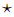mb = τ (a) mν (b), for all a, b ∈ B and all m ∈ τ M ν= M. If τ or ν is the identity map of B, then we write M ν orτ M, respectively. The enveloping algebra of an algebra B is the tensor product Be = B ⊗ Bop, where Bop is the opposite algebra of B. Bimodules over B are essentially the same as modules over the enveloping algebra of B. An algebra B is said to be homologically smooth, if as an Be -module, B has a projective resolution with finite length and such that every term in the projective resolution is finitely generated.

Definition 2.3. A graded algebra B is called skew Calabi-Yau of dimension d, if

• (i) B is homologically smooth, and

• (ii) there exists an algebra automorphism ν of B such that

as Be -modules, for some integer l.

The automorphism ν is called the Nakayama automorphism of B. If, in addition, Be is isomorphic to B as Be -modules, or equivalently, ν is inner, then B is called Calabi-Yau of dimension d.

Next, we recall the definition of our objects of interest introduced by Gallego and Lezama .

Definition 2.4. Let R and A be rings. We say that A is a skew P BW extension over R, if the following conditions hold:

• (i) R (the subring of coefficients) is a subring of A sharing the same identity element.

• (ii) There exist finitely many elements x1, . . . , xn ∈ A such that A is a left free Rmodule, with basis the set of standard monomials Mon(A) := {x α: = x 1α1 · · · x nα n | α = (α1, . . . , αn) ∈n}. Moreover, x 01 · · · x 0n: = 1 ∈ Mon(A).

• (iii) For every 1 ≤ i ≤ n and any r ∈ R \ {0}, there exists an element ci,r ∈ R \ {0} such that xir − ci,rxi ∈ R.

• (iv) For 1 ≤ i, j ≤ n, there exists di,j ∈ R \ {0} such that

If A is a skew PBW extension over R, then we denote it by A := σ(R){x1, . . . , xn}. For X = x α = x 1α1 · · · x nαn ∈ Mon(A), deg(X) = α1 + · · · + αn.

The notation and the name of the skew PBW extensions are due to the following proposition.

Proposition 2.5 (, Proposition 3). Let A = σ(R){x1, . . . , xn} be a skew P BW extension of R. For each 1 ≤ i ≤ n, there exists an injective endomorphism σi: R → R and a σi-derivation δi: R → R such that xir = σi(r)xi + δi(r), where r ∈ R.

From now on, σi and δi are the injective endomorphisms and σi-derivations, respectively, as in Proposition 2.5. A skew PBW extension A is called bijective, if σi is bijective and di,j is invertible, for any 1 ≤ i < j ≤ n. A is called quasi-commutative, if the conditions (iii) and (iv) in Definition 2.4 are replaced by the following:

(iii’) for each 1 ≤ i ≤ n and every r ∈ R \ {0}, there exists ci,r ∈ R \ {0} such that xir = ci,rxi ;

(iv’) for any 1 ≤ i, j ≤ n, there exists di,j ∈ R \ {0} such that xjxi = di,jxixj .

Different examples of skew PBW extensions can be found in Lezama et al.,  and Suárez . Ring and theoretic properties of these objects have been studied by the authors in [28, 30, 31].

The next result is key to define graded skew P BW extensions (see Definition 2.4).

Proposition 2.6 (, Proposition 2.7(ii)). Let A = σ(R){x1, . . . , xn} be a bijective skew P BW extension over an-graded algebra R =m≥0 Rm. If the following conditions hold,

• (i) σiis a graded ring homomorphism and δi : R(−1) → R is a graded σi-derivation, for all 1 ≤ i ≤ n, and

• (ii) xjxi − di,jxixj ∈ R2 + R1x1 + · · · + R1xn, as in (1) and di,j ∈ R0,

then A is an-graded algebra with graduation given by A =p≥0 Ap, where, for p ≥ 0, Ap is the-spacegenerated by the set

Definition 2.7 (, Definition 2.6). Let A = σ(R){x1, . . . , xn} be a bijective skew P BW extension over an-graded algebra R =m≥0 Rm. If A satisfies the conditions (i) and (ii) established in Proposition 2.6, then we say that A is a graded skew PBW extension over R.

Remark 2.8. If A = σ(R){x1, . . . , xn} is a quasi-commutative skew PBW extension over a ring R, then A is isomorphic to an iterated Ore extension of endomorphism type (, Theorem 2.3). Nevertheless, skew PBW extensions of endomorphism type are more general than iterated Ore extensions. With the aim of illustrating the differences between these structures, we consider the situations with two and three indeterminates.

If we take the iterated Ore extension of endomorphism type R[x; σx][y; σy], by definition (see Ore  or Goodearl and Warfield ), for any element r ∈ R, we have the following relations: xr = σx(r)x, yr = σy(r)y, and yx = σy(x)y. On the other hand, if we have σ(R){x, y} a skew P BW extension of endomorphism type over R, then for any r ∈ R, by Definition 2.4, we have the relations xr = σ1(r)x, yr = σ2(r)y, and yx = d1,2xy + r0 + r1x + r2y, for some elements d1,2, r0, r1 and r2 belong to R. When we compare the defining relations of both algebraic structures, it is clear which one of them is more general.

Now, if we have the iterated Ore extension R[x; σx][y; σy][z; σz], then for any r ∈ R, xr = σx(r)x, yr = σy(r)y, zr = σz(r)z, yx = σy(x)y, zx = σz(x)z, zy = σz(y)z. On the other hand, for the skew PBW extension of automorphism type σ(R){x, y, z}, we have the relations given by xr = σ1(r)x, yr = σ2(r)y, zr = σ3(r)z, yx = d1,2xy + r0 + r1x + r2y + r3z, zx = d1,3xz + r ´0 + r ´ 1x + r ´ 2 y + r ´ 3z, and zy = d2,3yz + r´´0 + r´´1x + r´´2y + r ´´3 z, for some elements d1,2, d1,3, d2,3, r0, r0 0 , r´´0 , r1, r´1 , r´´1 , r2, r´2 , r´´2 , r3, r´3 , r´´3 of R. Of course, as the number of indeterminates increases, the differences between both algebraic structures are more remarkable.

As we can expect from these facts, graded iterated Ore extensions are strictly contained in graded skew PBW extensions, see Suárez , Remark 2.11. Examples of graded skew PBW extensions can be found in Suárez et al., [9, 35, 36].

The following proposition contains several results about skew P BW extensions and it will be useful in the next section.

Proposition 2.9. Let A = σ(R){x1, . . . , xn} be a graded skew P BW extension.

• (i) If R is graded Noetherian, then A is graded Noetherian (, Proposition 2.7).

• (ii) If A is quasi-commutative, then R is Koszul if and only if A is Koszul (, Proposition 3.3).

• (iii) If R is Koszul finitely presented, then A is Koszul (, Theorem 5.5).

• (iv) If R is Artin-Schelter regular and A is quasi-commutative, then A is Artin-Schelter regular (, Theorem 3.6).

• (v) If R is a finitely presented connected Auslander-regular algebra, then A is ArtinSchelter regular (, Proposition 3.5).

• (vi) If A is quasi-commutative, R is connected and skew Calabi-Yau of dimension d, then A is skew Calabi-Yau of dimension d + n (, Theorem 4.5 (i)).

• (vii) If R is finitely presented connected Auslander-regular, then A is skew Calabi-Yau (, Theorem 4.5 (ii)).

Zhang and Zhang in  introduced a special class of algebras called double Ore extensions.

Definition 2.10 (, Definition 1.3). Let R be an algebra and A be another algebra containing R as a subring.

• (1) A is a right double Ore extension of R, if the following conditions hold:

• (i) A is generated by R and two new variables x1 and x2.

• (ii) The variables {x1, x2} satisfy the relation

where p12, p11and τ1, τ2, τ0 ∈ R.

• (2) A is a left double Ore extension of R, if the following conditions hold:

• (ib) A is generated by R and two new variables x1 and x2.

• (iib) The variables {x1, x2} satisfy the relation

where p´12, p´11and τ´1, τ ´2 , τ ´0 ∈ R.

• (iii) A is a double Ore extension, if it is left and right double Ore extension of R with the same generating set {x1,x2}.

• (iv) A is a graded right (left) double Ore extension, if all relations of A are homogeneous with assignment deg(x1) = deg(x2) = 1.

Let P denote the set of scalar parameters {p12, p11} and let τ denote the set {τ1, τ2, τ0}. We call P the parameter and τ the tail (see , p. 2671). By considering the datum P, Zhu et al.,  defined a matrix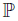∈ M2() in the following way:

By using the ideas given in , p. 2671, we rewrite the condition (3) above as follows:

Writing

it is clear that σ is a-linear map from R to M2(R), δ is a-linear map from R to the column R-module R⊕2: =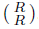, and σ and δ are uniquely determined. In this way, equation (5) can also be written as

which is a generalization of the multiplication in an Ore extension.

Let A be a right double Ore extension of R. Having in mind Definition 2.10, all symbols of {P, σ, δ, τ} are now defined. When no confusion arises, a right double Ore extension or a double Ore extension A is also denoted by A = RP [x1, x2; σ, δ, τ ]. Let A = RP [x1, x2; σ, δ, τ ] be a right double Ore extension. If δ is a zero map and τ consists of zero elements, then the right double Ore extension is denoted by RP [x1, x2; σ] and is called a trimmed right double Ore extension (see [43, Convention 1.6]).

Lemma 2.11 (, Lemma 1.7). If A = RP [x1, x2; σ, δ, τ ] is a right double Ore extension of R with {σ, δ} defined as in (5), then the following holds:

• (i) σ : R → M2(R) is an algebra homomorphism.

• (ii) δ : R → R⊕2 is a σ-derivation.

• (iii) If σ : R → M2(R) is an algebra homomorphism and δ: R → R⊕2 is a σ-derivation, then (5) holds for all elements r ∈ R if and only if it holds for a set of generators.

By Lemma 2.11, when A = RP [x1, x2; σ, δ, τ ] is a right double Ore extension, then σ is an algebra homomorphism and δ a σ-derivation. We now call σ a homomorphism, δ a derivation. Note that homomorphism σ is not surjective, so it cannot be invertible in the usual sense. Zhang and Zhang  defined invertibility of σ in right double Ore extensions.

Definition 2.12 (, Definition 1.8). Let σ : R → M2(R) be an algebra homomorphism. We say that σ is invertible, if there exists an algebra homomorphism

satisfying the following conditions:

for all r ∈ R. Equivalently,

where • is the multiplication of the matrix algebra M2(EndK(R)). The multiplication of EndK(A) is the composition of-linear maps. The map φ is called the inverse of σ.

Let A = RP [x1, x2; σ, δ, τ ] be a right double Ore extension. Zhang and Zhang  introduced the-linear map detP σ : R → R of σ :=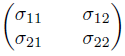, which is called the P-determinant.

Definition 2.13. If A = RP [x1, x2; σ, δ, τ ] is a right double Ore extension, then the P-determinant of σ is defined to be

Remark 2.14. If P = {1, 0}, then det P σ = σ22σ11 − σ12σ21. If p12 ‡ 0, then det P σ = −p−112 p11σ11σ22 − p −112 σ21σ12 + σ11σ22 (see , p. 2677). Moreover, det P σ is an algebra endomorphism of R (see Zhang , Proposition 2.1).

Let V be a finite-dimensional vector space and T(V ) be the tensor algebra with the usual grading. A connected graded algebra B = T(V )/hRi is quadratic, if R is a subspace of V ⊗2. The homogeneous dual of B is then defined as B! = T(V )/{R}, where V is the dual space of V and R = {λ ∈ V ⊗ V | λ(r) = 0, for all r ∈ R}. If B = T(V )/{R} is a Koszul algebra, then its Yoneda algebra is defined as E(B) :=i∈N ExtiB (,) which is isomorphic to B! . For a graded automorphism ν of B, we define a map ν : V → V by ν (f)(x) = f(ν(x)), for each f ∈ V and x ∈ V . Note that ν induces a graded automorphism of B! because ν is assumed to preserve the relation space R. We still use the notation ν for this algebra automorphism (see Zhun , p. 559).

Proposition 2.15 (, Proposition 1.11). Let B be a Koszul Artin-Schelter regular algebra with global dimension d. If ν is a graded automorphism of B and ν is its corresponding dual graded automorphism of the dual algebra B! , then there exists a unique c in the multiplicative group\ {0} such that ν (u) = cu, for all u ∈ ExtdA (,).

In  the homological determinant of a graded automorphism of an Artin-Schelter Gorenstein algebra was defined to study the noncommutative invariant theory. Since every Artin-Schelter Gorenstein algebra is Artin-Schelter regular, then for an Artin-Schelter Gorenstein algebra B, its homological determinant, denoted hdet, it is a homomorphism from the graded automorphism group of B to the multiplicative group\ {0}. The precise definition and its application can be found in [16, 27]. We use Proposition 2.15 to define the homological determinant.

Definition 2.16 (, Definition 1.5). Let B be a Koszul Artin-Schelter regular algebra and let ν be a graded automorphism of B. The homological determinant hdet(ν) of σ is defined as hdet(ν) := c, where c is as in Proposition 2.15.

Zhu et al.,  defined the homological determinant of σ, denoted hdetM, in the following way:

Definition 2.17 (, Definition 2.5). Let B be a Koszul Artin-Schelter regular algebra. Let σ : B → M2(B) be an algebra homomorphism and let σ ∗ : B! → M2(B! ) be the dual homomorphism of algebras. The homological determinant of σ, denoted by hdetM(σ), is defined to be

for W, X, Y, Zsatisfying σ (x0) =, where x0 is a base element of the highest nonzero component B!d , which is 1-dimensional-space, of B! .

Some properties, applications and examples of the homological determinant of σ can be found in Zhun et al., .

3. Main results

In this section, we present the new results for graded skew P BW extensions concerning ring-theoretic properties.

Theorem 3.1. If A = σ(R){x1, . . . , xn} is a graded skew P BW extension over a finitely presented Koszul Auslander-regular algebra R, then the homological determinant of the Nakayama automorphism of A is 1.

Proof. Since R is finitely presented Koszul, Proposition 2.9(iii) asserts that A is Koszul. Moreover, since R is Koszul, it is known that R is quadratic, and therefore connected. By assumption that R is Auslander-regular, and by Proposition 2.9(v), we have that A is Artin-Schelter regular. Note that by Proposition 2.9(vii), A is skew Calabi-Yau and there is the Nakayama automorphism ν of A. Now, since R is Auslander-regular, necessarily it is Noetherian. Thus, by Proposition 2.9(i), A is Noetherian. Finally, by Reyes et al., , Theorem 6.3, hdet(ν) = 1.

Corollary 3.2. If A = σ(R){x1, . . . , xn} is a graded skew P BW extension over the commutative polynomial ring R =[t1, . . . , tm], then the homological determinant of the Nakayama automorphism of A is 1.

Example 3.3. Some algebras which are graded skew P BW extensions over commutative polynomial rings are the following:

1. Let g be a finite dimensional Lie algebra over k with basis x1, . . . , xn and U(g) its enveloping algebra. The homogenized enveloping algebra of g is A(g) := T(gkz)/ hRi, where T(gkz) is the tensor algebra, z is a new indeterminate, and R is spanned by the union of sets {zx − xz | x ∈ g} and {xy − yx − [x, y]z | x, y ∈ g}. The algebra A(g) is a skew P BW extension over k[z] (c.f. Suárez et al., , Section 2.2).

2. The Jordan Algebra introduced by Jordan  is the free k-algebra J defined by J := k{x, y}/{yx − xy − y2 }. It is immediate that this algebra is not a skew polynomial ring of automorphism type but an easy computation shows that J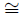σ(k[y]){x}.

3. Diffusion algebras arose in physics as a possible way to understand a large class of 1-dimensional stochastic process, see . A diffusion algebra A with non-zero parameters aij in the complex numbers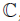, is an algebra overgenerated by variables x1, . . . , xn subject to relations aijxixj −bijxjxi = rjxi −rixj , whenever i < j, bij , ri, for all i < j, such that the indeterminates x’s form a-basis of the algebra A. In the applications to physics the parameters aij are strictly positive reals and the parameters bij are positive reals as they are unnormalised measures of probability. One can see that these algebras are not skew polynomial rings over[x1, . . . , xn] but are skew P BW extensions over this ring (see , Section 5.3).

Theorem 3.4. Let A = σ(R){x1, x2} be a graded skew P BW extension over a connected algebra R.

(i) A is a connected graded double Ore extension RP [x1, x2; σ, δ, τ ], where

with σ1, σ2, δ1and δ2 as in Proposition 2.5 and d1,2as in (1).

(ii) detP σ = σ2σ1, P = {c1,2, 0} and

(iii) σ is invertible with inverse

such that for all rR, the equation

holds for

(iv)

Proof. Suppose that A = σ(R){x1, x2} is a graded skew PBW extension of a connected algebra R. It is clear that A is a connected algebra. (i) Definition 2.4 (iii) establishes that for all r ∈ R \ {0}, there are elements c1,r, c2,r, c1,0, c2,0 ∈ R, with c1,r, c2,r ‡ 0, such that

In this way, for all r ∈ R, σ1(r) = c1,r, σ2(r) = c2,r, δ1(r) = c1,0, δ2(r) = c2,0, where σ1, σ2, δ1 and δ2 are as in Proposition 2.5. From , Theorem 3.2, we can assert that A is a connected graded double Ore extension RP [x1, x2; σ, δ, τ ]. (ii) Comparing relations (5) and (8), we have that

Therefore,

Hence, det P σ = σ2σ1. Now, from Definition 2.4 (iv), we know that there exists an element c1,2 ∈ R \ {0} such that

with r0, r1, r2 ∈ R. By assumption, A is graded, so deg(r0) = 2, deg(r1) = deg(r2) = 1 and deg(c1,2) = 0. Since R is connected, R0 =, whence c1,2\ {0}. Comparing (2) and (9), we have that p12 = c1,2, p11 = 0, τ1 = r1, τ2 = r2, τ0 = r0. Thus P = {c1,2, 0} and

(iii) By (i) and Zhun et al., , Lemma 1.8(1), we have that σ is invertible with its inverse given by

such that the equation r(x1x2) = (x1x2)φ(r) + δ ´ (r) holds for some

Then rx1 = x1φ11(r) + x2φ21(r) + δ´1 (r) and rx2 = x1φ12(r) + x2φ22(r) + δ´2 (r). Now, since A = σ(R){x1, x2} is a skew PBW extension, then

and

By Definition 2.4(ii), in Equation 10 we have that

and

Since A is a graded skew PBW extension, then A is bijective and therefore σ1 and σ2 are bijective. So, by Equation (12), σ −1 1 = φ11 and by Equation (13) we have that φ21 = 0 and −δ111) = −δ11−1 ) = δ´1 . Analogous to the above reasoning we have φ12 = 0 and −δ22−1 ) = δ´2 . (iv) It follows from (i) and , Proposition 1.14(a).

Theorem 3.5. Let R be a Koszul Artin-Schelter regular algebra with Nakayama automorphism ν and let A = σ(R){x1, x2} be a graded quasi-commutative Calabi-Yau skew P BW extension. Then

where σ1, σ2 are as in Proposition 2.5 and d1,2 is as in (1).

Proof. Since A is a connected graded quasi-commutative skew PBW extension of R then by , Corollary 3.3, we have that A is a connected graded trimmed right double Ore extension of R, of the form A = RP [x1, x2; σ]. By Theorem 3.4, det P σ = σ2σ1 and

By the assumption, R is Artin-Schelter regular and A is quasi-commutative, so Proposition 2.9(iv) implies that A is Artin-Schelter regular. Since R is Koszul then by Proposition

2.9(iii) we have that A is Koszul. Since A is Calabi-Yau, then by Zhun , Theorem 3.12,

i.e.,

According to the calculations presented in , Example 2.7,

which implies that hdet(σ1) = c1,2, and hdet(σ2) =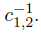Corollary 3.6. If A = σ(R){x1, x2} is a graded quasi-commutative skew P BW extension over a Koszul Artin-Schelter regular algebra R with Nakayama automorphism ν, then

• (i) The Nakayama automorphism µ of A is given by

• (ii) A is Calabi-Yau if and only if ν = σ2σ1 and

Proof. From the proof of Theorem 3.5, we know that A is a Koszul Artin-Schelter regular connected graded trimmed right double Ore extension of R given by A = RP [x1, x2; σ]. (i) By , Proposition 3.11, the Nakayama automorphism µ of A is given by

Now, from Theorem 3.4, we have that

By replacing (16) in (15), we obtain (14).

(ii) If A is Calabi-Yau, then by Theorem 3.5, ν = σ2σ1 and

Conversely, by replacing ν = σ2σ1 and

in (14), we have that µ is the identity map of A. Definition 2.3 asserts that A is CalabiYau.

Acknowledgments

The third named author was supported by the research fund of Departamento de Matemáticas, Universidad Nacional de Colombia - Sede Bogotá, HERMES CODE 41535.

References

 Acosta J.P. and Lezama O., “Universal property of skew PBW extensions”, Algebra and Discrete Math., 20 (2015), No. 1, 1-12. [ Links ]

 Artamonov V.A., “Derivations of skew PBW extensions”, Commun. Math. Stat., 3 (2015), No. 4, 449-457. doi: 10.1007/s40304-015-0067-9 [ Links ]

 Artin M. and Schelter W.F., “Graded algebras of global dimension 3”, Adv. Math., 66 (1987), 171-216. doi: 10.1016/0001-8708(87)90034-X [ Links ]

 Backelin J. and Fröberg R., “Koszul algebras, Veronese subrings and rings with linear resolutions”, Rev. Roumaine Math. Pures Appl., 30 (1985), No. 2, 85-97. [ Links ]

 Bell A. and Goodearl K., “Uniform rank over differential operator rings and PoincaréBirkhoff-Witt extensions”, Pacific J. Math., 131 (1988), No. 1, 13-37. [ Links ]

 Carvalho P., Lopes S. and Matczuk J., “Double Ore extensions versus iterated Ore extensions”, Comm. Algebra, 39 (2011), No. 8, 2838-2848. doi: 10.1080/00927872.2010.489532 [ Links ]

 Fajardo W., Gallego C., Lezama O., Reyes A., Suárez H., and Venegas H., Skew P BW Extensions: Ring and Module-theoretic Properties, Matrix and Gröbner Methods, and Applications, Springer Nature, vol. 28, 2020. [ Links ]

 Gallego C. and Lezama O., “Gröbner bases for ideals of σ-PBW extensions”, Comm. Algebra, 39 (2011), No. 1, 50-75. doi: 10.1080/00927870903431209 [ Links ]

 Gómez J.Y. and Suárez H., “Double Ore extensions versus graded skew PBW extensions”, Comm. Algebra, 48 (2020), No. 1, 185-197. [ Links ]

 Goodearl K.R. and Warfield R.B. Jr., An Introduction to Noncommutative Noetherian Rings, Cambridge University Press, 2nd ed., vol. 61, Cambridge, 2004. doi: 10.1017/CBO9780511841699 [ Links ]

 Hashemi E., Khalilnezhad K. and Alhevaz A., “(Σ, ∆)-Compatible skew PBW extension ring”, Kyungpook Math. J., 57 (2017), No. 3, 401-417. doi: 10.5666/KMJ.2017.57.3.401 [ Links ]

 Hashemi E., Khalilnezhad K. and Alhevaz A., “Extensions of rings over 2-primal rings”, Le Matematiche, 54 (2019), No. 1, 141-162. doi: 10.4418/2019.74.1.10 [ Links ]

 Hashemi E., Khalilnezhad K. and Ghadiri Herati M., “Baer and quasi-Baer properties of skew PBW extensions”, J. Algebraic Systems, 7 (2019), No. 1, 1-24. [ Links ]

 Isaev A.P., Pyatov P.N. and Rittenberg V., “Diffusion algebras”, J. Phys. A., 34 (2001), No. 29, 5815-5834. doi: 10.1088/0305-4470/34/29/306 [ Links ]

 Jordan D., “The graded algebra generated by two Eulerian derivatives”, Algebr. Represent. Theory, 4 (2001), No. 3, 249-275. doi: 10.1023/A:1011481028760 [ Links ]

 Jorgensen P. and Zhang J.J., “Gourmet’s Guide to Gorensteinness”, Adv. Math., 151 (2000), No. 2, 313-345. doi: 10.1006/aima.1999.1897 [ Links ]

 Lezama O., “Computation of point modules of finitely semi-graded rings”, Comm. Algebra, 48 (2020), No. 2, 866-878. doi: 10.1080/00927872.2019.1666404 [ Links ]

 Lezama O. and Gallego C., “d-Hermite rings and skew PBW extensions”, São Paulo J. Math. Sci., 10 (2016), No. 1, 60-72. doi: 10.1007/s40863-015-0010-8 [ Links ]

 Lezama O. and Gómez J., “Koszulity and Point Modules of Finitely Semi-Graded Rings and Algebras”, Symmetry, 11 (2019), No. 7, 1-22. doi: 10.3390/sym11070881 [ Links ]

 Lezama O. and Reyes A., “Some homological properties of skew PBW extensions”, Comm. Algebra, 42 (2014), No. 3, 1200-1230. doi: 10.1080/00927872.2012.735304 [ Links ]

 Lezama O. and Venegas C., “Center of skew PBW extensions”, Internat. J. Algebra Comput., 30 (2020), No. 8, 1625-1650. doi: 10.1142/S0218196720500575 [ Links ]

 Liu Y. and Ma W., “Nakayama automorphism of Ore extensions over polynomial algebras”, Glasg. Math. J., 62 (2020), No. 3, 518-530. doi: 10.1017/s0017089519000259 [ Links ]

 Liu Y., Wang S. and Wu Q.-S., “Twisted Calabi-Yau property of Ore extensions”, J. Noncommut. Geom., 8 (2014), No. 2, 587-609. doi: 10.4171/JNCG/165 [ Links ]

 Lu J., Mao X. and Zhang J.J., “Nakayama automorphism and applications”, Trans. Amer. Math. Soc., 369 (2017), No. 4, 2425-2460. doi: 10.1090/tran/6718 [ Links ]

 Ore O., “Theory of non-commutative polynomials”, Ann. Math, 34 (1933), No. 3, 480-508. doi: 10.2307/1968173 [ Links ]

 Polishchuk A. and Positselski L., Quadratic algebras, American Mathematical Society, vol. 37, Providence, RI, 2005. doi: 10.1090/ulect/037 [ Links ]

 Reyes M., Rogalski D. and Zhang J.J., “Skew Calabi-Yau algebras and homological identities”, Adv. Math., 264 (2014), 308-354. doi: 10.1016/j.aim.2014.07.010 [ Links ]

 Reyes A. and Suárez H., “Armendariz property for skew P BW extensions and their classical ring of quotients”, Rev. Integr. Temas Mat., 34 (2016), No. 2, 147-168. doi: 10.18273/revint.v34n2-2016004 [ Links ]

 Reyes A. and Suárez H., “Enveloping Algebra and Skew Calabi-Yau algebras over Skew Poincaré-Birkhoff-Witt extensions”, Far East J. Math. Sci., 102 (2017), No. 2, 373-397. doi 10.17654/MS102020373 [ Links ]

 Reyes A. and Suárez H., “Radicals and Köthe’s conjecture for skew PBW extensions”, Commun. Math. Stat., (2019). doi: 10.1007/s40304-019-00189-0 [ Links ]

 Reyes A. and Suárez H., “Skew Poincaré-Birkhoff-Witt extensions over weak compatible rings”, J. Algebra Appl., 19 (2020), No. 12, 21. doi: 10.1142/S0219498820502254 [ Links ]

 Reyes A. and Suárez H., “Skew Poincaré-Birkhoff-Witt extensions over weak zip rings”, Beitr. Algebra Geom., vol. 60 (2019), No. 2, 197-216. doi: 10.1007/s13366-018-0412-8 [ Links ]

 Shen Y. and Lu D.-M., “Nakayama automorphisms of P BW deformations and Hopf actions”, Sci. China Math., 59 (2016), No. 4, 661-672. doi: 10.1007/s11425-015-5077-2 [ Links ]

 Shen Y., Zhou G-S. and Lu D-M., “Nakayama automorphisms of twisted tensor products”, J. Algebra, 504 (2018), 445-478. doi: 10.1016/j.jalgebra.2018.02.025 [ Links ]

 Suárez H., “Koszulity for graded skew PBW extensions”, Comm. Algebra, 45 (2017), No. 10, 4569-4580. doi: 10.1080/00927872.2016.1272694 [ Links ]

 Suárez H., Lezama O. and Reyes A., “Calabi-Yau property for graded skew PBW extensions”, Rev. Colombiana Mat., 51 (2017), No. 2, 221-238. doi: 10.15446/recolma.v51n2.70902 [ Links ]

 Suárez H. and Reyes A., “Koszulity for skew PBW extensions over fields”, JP J. Algebra Number Theory Appl., 39 (2017), No. 2, 181-203. doi: 10.17654/NT039020181 [ Links ]

 Suárez H. and Reyes A., “A generalized Koszul property for skew PBW extensions”, Far East J. Math. Sci., 101 (2017), No. 2, 301-320. doi: 10.17654/MS101020301 [ Links ]

 Suárez H. and Reyes A., “Nakayama automorphism of some skew PBW extensions”, Ingeniería y Ciencia, 15 (2019), No. 29, 157-177. doi: 10.17230/ingciencia.15.29.6 [ Links ]

 Tumwesigye A.B., Richter J. and Silvestrov S., Centralizers in PBW extensions, Springer, vol. 317, Cham, 2020. doi: 10.1007/978-3-030-41850-2-20 [ Links ]

 Wu Q. and Zhu C., “Skew group algebras of Calabi-Yau algebras”, J. Algebra, 340 (2011), 53-76. doi: 10.1016/j.jalgebra.2011.05.027 [ Links ]

 Zambrano B.A., “Poisson brackets on some skew PBW extensions”, Algebra Discrete Math., 29 (2020), No. 2, 277-302. [ Links ]

 Zhang J.J. and Zhang J., “Double Ore extensions”, J. Pure Appl. Algebra, 212 (2008), No. 12, 2668-2690. doi: 10.1016/j.jpaa.2008.05.008 [ Links ]

 Zhang J.J. and Zhang J., “Double extension regular algebras of type (14641)”, J. Algebra, 322 (2009), No. 2, 373-409. doi: 10.1016/j.jalgebra.2009.03.041 [ Links ]

 Zhu C., Van Oystaeyen F. and Zhang Y., “Nakayama automorphisms of double Ore extensions of Koszul regular algebras”, Manuscripta Math., 152 (2017), No. 3-4, 555-584. doi: 10.1007/s00229-016-0865-8 [ Links ]

*Dedicated to professor Oswaldo Lezama for his brilliant academic careerat the Universidad Nacional de Colombia - Sede Bogotá

Received: September 05, 2020; Accepted: December 09, 2020

** Corresponding author. mareyesv@unal.edu.coEsta obra está bajo una Licencia Creative Commons Atribución 4.0 Internacional.